# OCR Machine Learning: Retrieving Information from OCR Text

by Ivan Ozhiganov

14 Dec 2016

Nowadays automated OCR systems demonstrate fairly high performance in character recognition. However, research engineers cannot achieve 100% precision. For this reason, the issues of information search and fixing mistakes are still a high priority in the scientific and R&D community. Usually, for solving these problems, text corrections are applied using the vocabulary of the text language or the language model. In particular, language models word2vec and siamese LSTM can be used for information retrieval.

We looked at the problem of information retrieval from OCR text within a project that worked on developing receipt recognition system. Our research became a new stage on the way to the growth of recognition performance.

The goal of our research is to increase the retrieval effectiveness in the presence of noisy text.

## Research Overview

• Identifying the problem of information retrieval from OCR text
• Applying heuristic algorithm
• Choosing a method of machine learning
• Using a fully convolutional neural network for problem-solving
• Applying a recurrent neural network LSTM for problem-solving

## Implementation

### 1. Identifying the problem of information retrieval from OCR text

After applying the OCR system to receipt recognition, we received a dataset of recognized texts with some distortions. And we continued with information retrieval from this OCR text.

Our main task is to extract specific data from the text: the shopping list, ITN, date, etc. The text in images was recognized with mistakes. It is shown on the pictures of receipts and in the recognized text. Take a look at image 1 and image 2. You can also see the distortions on images 3 and 4 with the section of purchases and a highlighted purchase.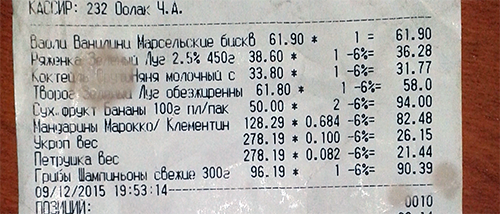##### Image 1: Receipt image with the text##### Image 2: An example of the recognized text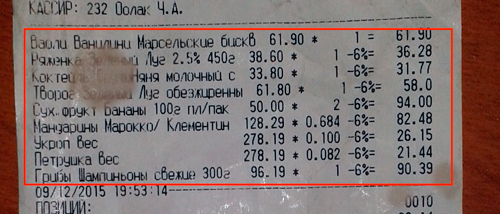##### Image 3: The section of purchases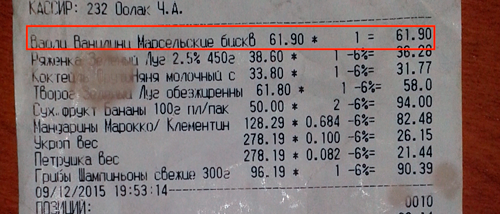### 2. Applying a heuristic algorithm

First we chose a heuristic algorithm for information retrieval from noisy text after OCR. Heuristics look like regular expressions and complex algorithmic constructions. We thought they would be perfect for the task of extracting the required data from the text. However, this approach turned out to be not flexible enough and very complicated in practice. While we were retrieving information, we found receipts that were much more complex for recognition, and that led us to writing more complex and clumsy heuristics.

Thus, we decided to consider some alternative options for achieving the main goal. For example, methods of machine learning.

### 3. Choosing a method of OCR machine learning

We’ve used machine learning methods several times during past research. We implemented experiments with convolutional neural networks for car license recognition and for face recognition. In addition to these projects, we took part in the competition for building the EEG signal classification.

The basis of machine learning is learning from examples. Imagine the model (for instance, LSTM network) that is learned from a large amount of manually marked examples. Then you can apply the trained model for marking new samples that were not included in the original dataset.

Compared to the heuristic algorithm, machine learning has many advantages:

• A more flexible approach
• The models learn relatively faster with new data
• A model can learn to use features that are not obvious to a man (for example, line-wrapping and less symbols without breaks in the next row)

We used two models of machine learning in our research — fully convolutional neural network and recurrent neural network LSTM. Let’s consider both of them and analyze the results of each.

### 4. Using a fully convolutional neural network for problem-solving

We tried to create a system for finding purchases in receipts using a fully convolutional neural network.##### Image 5: Applying fully convolutional network to find purchases

The purchases are marked by the symbol “_” that means the start of the purchase and the symbol “^” - the end of the purchase. Symbols “_” and “^” were put in the text manually. We expected that the neural network would learn on the basis of manually marked examples and find the purchases in new receipts that are not included in the training.

In general, a fully convolutional neural network is dedicated to image recognition. In order to apply the method of information retrieval through the model of the fully convolutional neural network, we designed a rule for coding text into the image.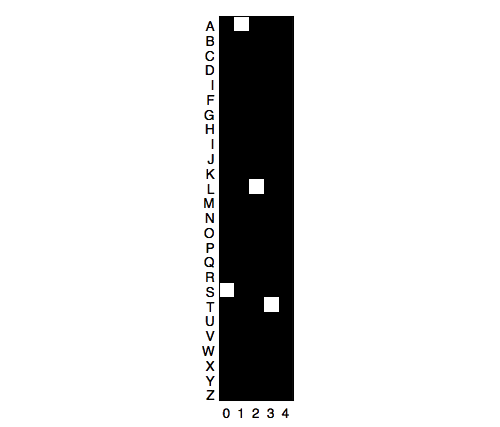##### Image 6: Coding word salt into the image

Coding was implemented according to the rule:

`Ii,j=1 if symbol with number i placed at j-position`
`Ii,j=0 otherwise`

Where Iij is a two-dimensional array of the image. The size of the Iij is equal to d x L (d is the length of the alphabet and L is the length of text).

For simplicity, there is a limited alphabet on image 6. We actually used the full alphabet including Russian and English letters, special symbols (spacebar, %, #, and others), numbers as well as an additional carriage return symbol for coding multiline text.

We got the following results:

We give the image Iij at the network input and receive at the output the image Oj with the size 1 x L such that:

`Oj=1 if the symbol in j-position belongs to the some purchase`
`Oj=0 if the symbol in j-position doesn’t belong to purchase`

The fully convolutional neural network transmits a two-dimensional image into a one-dimensional signal on the first layer. After that there are only transformations of one-dimensional signal taking place. You can see the work of the first layer on image 7.##### Image 7: The work of the first convolution layer

The red rectangle is an input image, the green rectangle is a convolution kernel, and the yellow squares are the output one-dimensional signal. The height of the convolution kernel equals to the height of the input image. Hence, at the output, we get an image with a 1-pixel height. In other words, it is a one-dimensional signal.

Next, this one-dimensional signal can be complemented by zeros (so called padding) on the left and right edges in a way that the signal length will be equal to the width of the input image. There is also the transformation of the one-dimensional signal to the one-dimensional signal on the second convolution layer. Besides the convolution kernel, there is one-dimensional as well. Take a look at the network architecture in image 8.##### Image 8: Fully convolutional network architecture

The max-pooling layers pool1 and pool2 had a stride equal to 1. On this basis, the scale of the signal didn’t change after the pooling. For training the network we wrote scripts in Python using Theano library.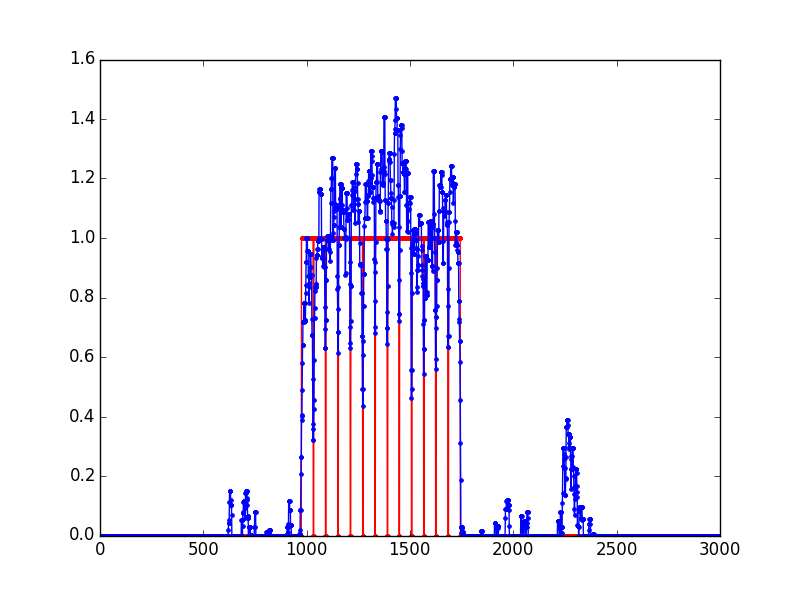##### Image 9: An example of the network output##### Image 10: Another example of the network output

The examples of network outputs on the test dataset were shown in images 9 and 10. The blue line demonstrates the network output. The red line shows what output should ideally be (ground truth). The x-axis is the number of symbol position. The y-axis is the probability of where the specific symbol is at some purchase. When the red line is on the level 1.0, it means that this is where a purchase is. There are short breakdowns to the value 0.0, which means carriage return symbols. They do not relate to any of the purchases.

We had several attempts of training the network with different parameters and various architecture. There was an overfitting in all the attempts: the value of loss in training dataset was approximately 0.002 whereas in test dataset it was 0.016. Due to the overfitting, we cannot achieve a sufficient result. According to the outcome of the experiments, the fully convolutional neural network sees the purchases, but not clearly. Besides, there was only a few receipts in the training dataset — 150. For these reasons, we didn’t finish the work with the fully convolutional neural network. The training dataset can be enlarged in future experiments using augmentation. Perhaps it can help to overcome the problem of overfitting and to obtain better results.

### 5. Applying a recurrent neural network LSTM for problem-solving

Recurrent neural networks are particularly well-suited for tasks where there are sequences. We have a sequence of symbols in our task, and that’s why we decided to try the model of recurrent neural networks.

Using the LSTM network we were trying to determine the complete section of purchases without defining specific positions in the receipt. The LSTM structure allows organizing long-term memory inside the network. It brings to the network an opportunity to use features that are relatively far apart for information retrieval.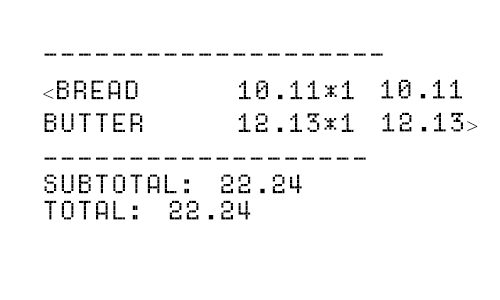##### Image 11: Highlighting the purchase section in the receipt

Here a symbol “<” means the start of the purchase section and “>” – the end of the purchase section. Symbols “<” and “>” were put in the text manually. The LSTM network architecture is shown in image 12.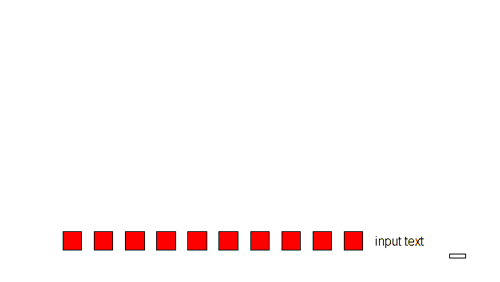##### Image 12: The LSTM network architecture

There are two LSTM layers. The first one goes along with the text from left to right. The second LSTM layer goes backward from right to left. The outputs of both LSTM layers are banded together and go into a dense layer. The labeling comes out the dense layer as a network response. LSTM network has a memory, hence the dense layer theoretically knows about all the symbols from the left and from the right in this architecture.

In the output layer, symbols are encoded by the one-hot encoding method:

`Ii=1 if the symbol number equals i`
`Ii=0 otherwise`

Where I is the input vector for one symbol.

In other words we input the sequence of vectors into the network, where the size of every vector is equal to the length of the alphabet and the number of vectors is equal to the number of symbols in the text. At the output, we get a sequence of two-dimensional vectors.

The network is trained according to the rule:

`O1=1.0 and O2=0.0 if the symbol doesn’t refer to the purchase section`
`O1=0.0 and O2=1.0 if the symbol refers to the purchase section`

Where O is the output vector for one symbol.

Thus, the value O2 after training on the testing stage is the probability of the symbol belonging to the purchase section. The condition O2>O1 is checked here. If the condition is not fulfilled, the network answer means that this symbol belongs to the purchase section. Otherwise, the symbol doesn’t belong to the purchase section.

Out training dataset included approximately 200 original receipts within the research. It was artificially augmented to 600 receipts. While augmenting the dataset, accidental errors can appear and random exchange of purchase sections between pairs of receipts takes place. We trained the LSTM network using the libraries Theano+Lasagne in Python and ADAM solver. You can see the example of loss evolution within training in image 13.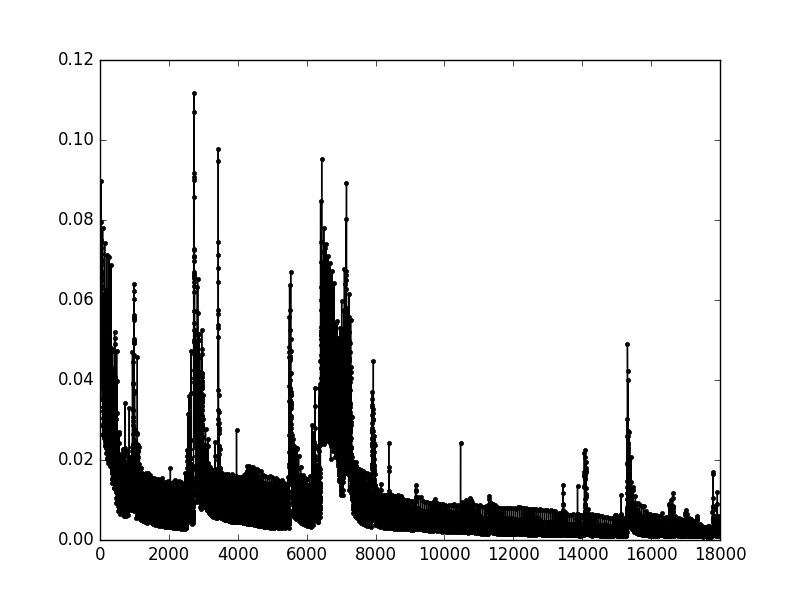##### Image 13: An example of loss evolution

The x-axis is the number of the solver step, the y-axis is the loss. It is obvious that the network is trained instable. We are going to handle this instability two ways:

• We reduce a learning rate

You can see the example of highlighting the section of purchases on the test image by the LSTM network. The test showed that the network labels the section of purchases almost precisely if the OCR text has not many errors.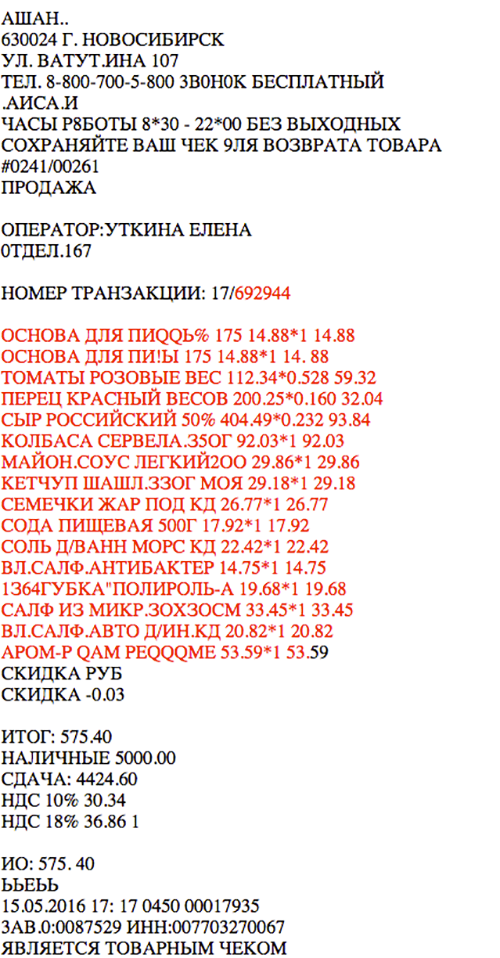##### Image 14: An example of highlighting the section of purchases on the test image

At the end, we came to the conclusion that the recurrent neural network LSTM proved to be efficient and can be successfully used for finding specific purchases in the receipt text. Hence, we are going to train the LSTM network for detection of specific purchases.

## The Outcome

Our research implies that to develop an algorithm for information retrieval from noisy text you need to apply several methods and compare them in practice. We implemented this successfully. After trying several approaches, we chose the most convincing — the algorithm of data retrieval using the recurrent neural network LSTM. In particular, a LSTM network helps to find information in the text with a template. All you need to provide reliable results is a large training dataset and stable learning process. Heuristic application at the output can become an additional advantage for increasing effectiveness.

• 0 SHARES